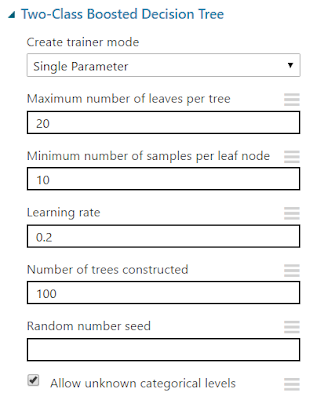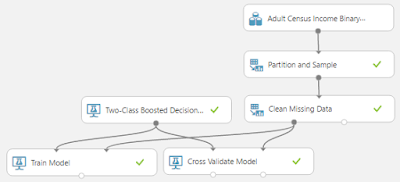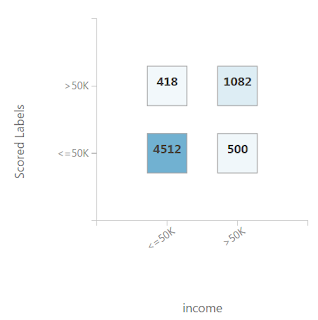## Monday, November 21, 2016

### Azure Machine Learning: Classification Using Two-Class Boosted Decision Tree

Today, we're going to continue our walkthrough of Sample 3: Cross Validation for Binary Classification Adult Dataset.  In the previous post, we walked through the initial data load, as well as the Two-Class Averaged Perceptron algorithm.  Now, we're going to walk through the next algorithm, Two-Class Boosted Decision Tree.  Let's start with a simple overview of the experiment.Sample 3: Cross Validation for Binary Classification Adult Dataset
The purpose of this data set is to take a dataset of Demographic data about individuals, and attempt to predict their income based on these factors.  Here's a snippet of that dataset.Adult Census Income Binary Classification Dataset (Visualize)Adult Census Income Binary Classification Dataset (Visualize) (Income)
If you want to learn more about the data import section of this experiment, check out the previous post.  Let's move on to the star of the show, Two-Class Boosted Decision Tree.  This is one of our favorite algorithms because it is incredibly simple to visualize, yet offers extremely powerful predictions.Two-Class Boosted Decision Tree
This algorithm doesn't just construct one tree, it constructs as many as you want (100 in this case).  What's extremely interesting about these additional trees is that they are not independent of their predecessors.  According to MSDN, "the second tree corrects for the errors of the first tree, the third tree corrects for the errors of the first and second trees, and so forth."  This means that our trees should get better as we increase our "Number of Trees Constructed" parameter.  Unfortunately, this would mean that trees later in the process have a much higher risk of "Overfitting" than trees earlier in the process.  "Overfitting" is a situation where the model has been trained so heavily that it can extremely accurately predict your training data, but be very poor at predicting new observations.  Fortunately, the algorithm accounts for this by not just taking the prediction from the final tree in the set.  It takes predictions from every tree and averages them together.  This greatly lessens the effect of "Overfitting" while still providing accurate predictions.

The "Maximum Number of Leaves per Tree" parameter allows us to set the number of times the tree can split.  It's important to note that splits early in the tree are caused by the most significant predictors, while splits later in the tree are less significant.  This means that the more leaves you have (and therefore more splits), the higher your chance of overfitting is.  This is why Validation is so important.

The "Minimum Number of Samples per Leaf Node" parameters allows us to set the significance level required for a split to occur.  With this value set at 10, the algorithm will only choose to split (this known as creating a "new rule") if at least 10 rows, or observations, will be affected.  Increasing this value will lead to broad, stable predictions, while decreasing this value will lead to narrow, precise predictions.

The "Learning Rate" parameter allows us to set how much difference we see from tree to tree.  MSDN describes this quite well as "the learning rate determines how fast or slow the learner converges on the optimal solution. If the step size is too big, you might overshoot the optimal solution. If the step size is too small, training takes longer to converge on the best solution."

Finally, this algorithm lets us select a "Create Trainer Mode".  This is extremely useful if we can't decide exactly what parameters we want.  We'll take more about parameter selection in a later post.  If you want to learn more about this algorithm, read here and here.  Let's visualize this tool.Two-Class Boosted Decision Tree (Visualize)
Just like with the Two-Class Averaged Perceptron algorithm, the visualization of the untrained model is not very informative.  Strangely enough, this visualization shows us the correct parameters, whereas the Two-Class Averaged Perceptron did not.  What would be far more interesting is if we could look at the trained tree.  In order to do this, we need to add a new tool to our experiment, Train Model.Condensed ExperimentTrain Model
The Train Model initialization is pretty simple.  All we need to do is select our variable of interest, which is "Income" in this case.  Let's take a look at the visualization.Train Model (Visualization)
As you can see, this visualization lets you look through all the trees created in the training process.  Let's zoom in on a particular section of the tree.Train Model (Visualization) (Zoom)
EDIT: At the time of writing this, there is a bug related to the display of predictions within Decision Trees.  Please see here for more details.

As you can see, each split in the tree relies on a single variable in a single expression, known as a predicate.  The first predicate says

marital-status.Married-civ-spouse <= 0.5

We've talked before about the concept of Dummy Variables.  When you pass a categorical variable to a numeric algorithm like this, it has to translate the values to numeric.  It does this by creating Dummy, or Indicator, Variables.  In this case, it created Dummy Variables for the "marital-status" variable.  One of these variables is "marital-status.Married-civ-spouse".  This variable takes a value of 1 if the observation has "marital-status = Married-civ-spouse" and 0 otherwise.  Therefore, this predicate is really just a numeric way of saying "Does this person have a Marital Status of "Married-Civ-Spouse".  We're not sure exactly what this means because this isn't our data set, but it's the most common variable in the dataset.  Therefore, it probably means being married and living together.

Under the predicate definition, we also see a value for "Split Gain".  This is a measure of how significant the split was.  A large value means a more significant split.  Since Google is our best friend, we found a very informative answer on StackOverflow explaining this.  You can read it here.

What we find very interesting about this tree structure is that it is not "balanced".  This means that in some cases, we can reach a prediction very quickly or very slowly depending on which side of the tree we are on.  We can see one prediction in Level 2 (the root level is technically considered Level 0.  This means that the 3rd level is considered Level 2).  We're not sure what causes the tree to choose whether to predict or split.  The MSDN article seems to imply that it's based on the combination of the "Minimum Number of Samples per Leaf Node" as well as some internal Information (or Split Gain) threshold.  Perhaps one of our readers can enlighten us about this.

Since we talked heavily about Cross-Validation in the previous post, we won't go into too much detail here.  However, it may be interesting to see the Contingency table to determine how well this model predicted our data.Contingency Table (Two-Class Boosted Decision Tree)Contingency Table (Two-Class Averaged Perceptron)
As you can see, the number of correct predictions for "Income <= 50k" is about the same between the two algorithms, but the Two-Class Boosted Decision Tree wins when it comes to the "Income > 50k" category.  We would need more analysis to make a sound decision, but we'll have to save that for a later post.  Thanks for reading.  We hope you found this informative.